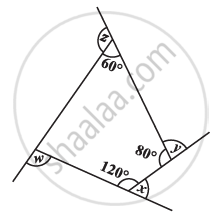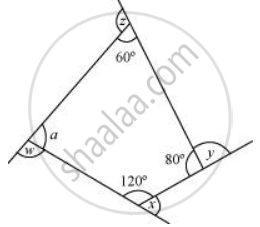# Find X + Y + Z + W - MathematicsFind x + y + z + w

#### SolutionSum of the measures of all interior angles of a quadrilateral is 360º. Therefore, in the given quadrilateral,

a + 60° + 80° + 120° = 360°

a + 260° = 360°

a = 100°

x + 120° = 180° (Linear pair)

x = 60°

y + 80° = 180° (Linear pair)

y = 100°

z + 60° = 180° (Linear pair)

z = 120°

w + 100° = 180° (Linear pair)

w = 80°

Sum of the measures of all interior angles = x + y + z + w

= 60° + 100° + 120° + 80°

= 360°

Concept: Angle Sum Property of a Quadrilateral
Is there an error in this question or solution?

#### APPEARS IN

NCERT Class 8 Maths